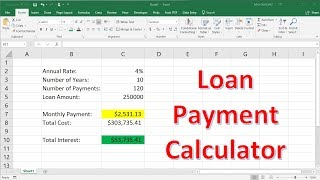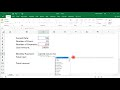# How To Calculate Loan Payments Using The PMT Function In Excel

Jun 19, 2018

### Thumbs### Description

This video tutorial explains how to calculate loan payments using the PMT function in excel. It explains how to calculate the monthly payment given the principal and annual interest rate. It also determines the total cost of the loan with the total interest paid over the lifetime of the loan.

Subscribe:

Excel Tutorial For Beginners:

Top 10 Excel Formulas:

Top 30 Excel Tips, Tricks, & Shortcuts:

How To Use VLookUp: